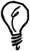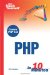# Numeric Data Types

You have already seen that PHP assigns a data type to each value and that the numeric data types are integer and double, for whole numbers.

To check whether a value is either of these types, you use the is_float and is_int functions. Likewise, to check for either numeric data type in one operation, you can use is_numeric.

The following example contains a condition that checks whether the value of \$number is an integer:

` \$number = "28"; if (is_int(\$number)) {   echo "\$number is an integer"; } else {   echo "\$number is not an integer"; } `

Because the actual declaration of that variable assigns a string valuealbeit one that contains a numberthe condition fails.

Although \$number in the previous example is a string, PHP is flexible enough to allow this value to be used in numeric operations. The following example shows that a string value that contains a number can be incremented and that the resulting value is an integer:

` \$number = "6"; \$number++; echo "\$number has type " . gettype(\$number); `

#### Understanding NULLs

The value NULL is a data type all to itselfa value that actually has no value. It has no numeric value, but comparing to an integer value zero evaluates to true:

` \$number = 0; \$empty=NULL; if (\$number == \$empty) {   echo "The values are the same"; } `Type Comparisons If you want to check that both the values and data types are the same in a condition, you use the triple equals comparison operator (===).Sams Teach Yourself PHP in 10 Minutes
ISBN: 0672327627
EAN: 2147483647
Year: 2005
Pages: 151
Authors: Chris Newman

Similar book on Amazon

flylib.com © 2008-2017.
If you may any questions please contact us: flylib@qtcs.net